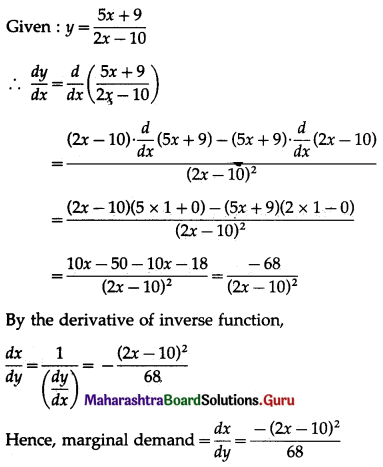Balbharati Maharashtra State Board 12th Commerce Maths Solution Book Pdf Chapter 3 Differentiation Ex 3.2 Questions and Answers.

## Maharashtra State Board 12th Commerce Maths Solutions Chapter 3 Differentiation Ex 3.2

1. Find the rate of change of demand (x) of a commodity with respect to price (y) if:

Question 1.
y = 12 + 10x + 25x2
Solution:
Given y = 12 + 10x + 25x2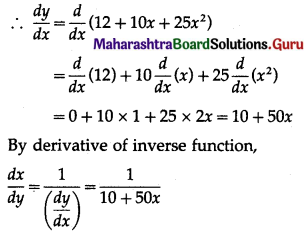Hence, the rate of change of demand (x) with respect to price (y) $$=\frac{d x}{d y}=\frac{1}{10+50 x}$$

Question 2.
y = 18x + log(x – 4)
Solution:
Given y = 18x + log (x – 4)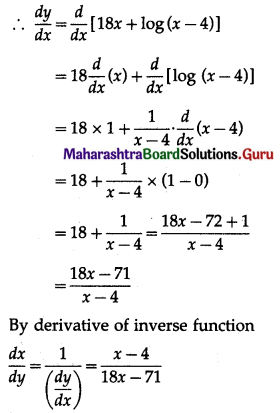Hence, the rate of change of demand (x) with respect to price (y) $$=\frac{d x}{d y}=\frac{x-4}{18 x-71}$$Question 3.
y = 25x + log(1 + x2)
Solution:
Given y = 25x + log(1 + x2)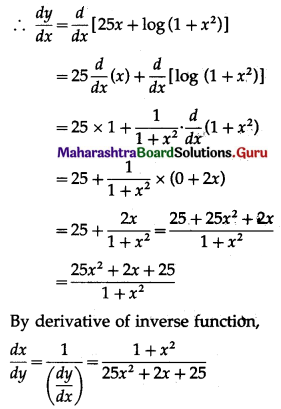Hence, the rate of change of demand (x) with respect to price (y) $$\frac{d x}{d y}=\frac{1+x^{2}}{25 x^{2}+2 x+25}$$

2. Find the marginal demand of a commodity where demand is x and price is y.

Question 1.
y = xe-x + 7
Solution: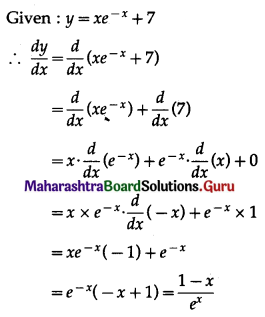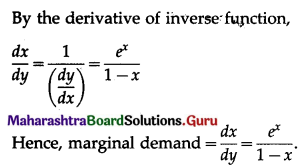Question 2.
y = $$\frac{x+2}{x^{2}+1}$$
Solution: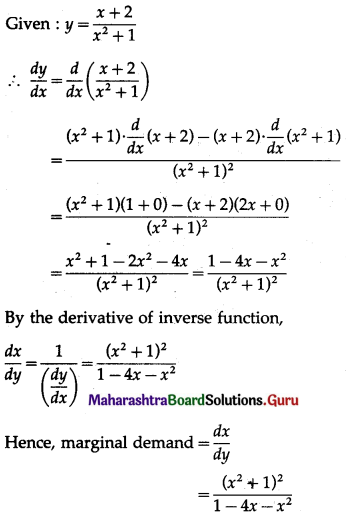Question 3.
y = $$\frac{5 x+9}{2 x-10}$$
Solution: